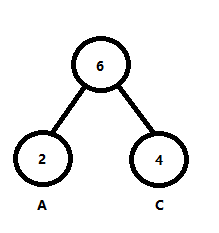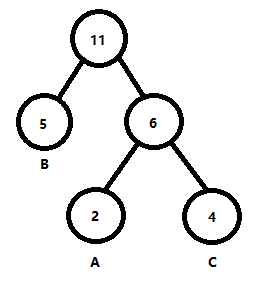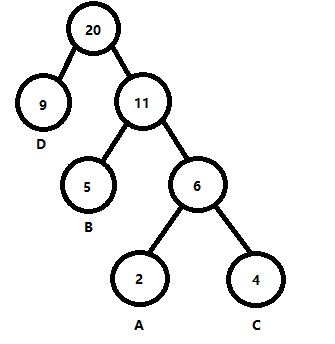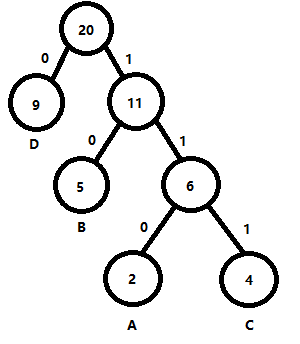# 哈夫曼树及哈夫曼编码（基于优先队列实现）

## 概念

### 基础

• 路径：从树中一个结点到另一个结点之间的分支
• 路径长度：路径上的分支数目称为路径长度
• 树的路径长度：从树根到每一结点的路径长度之和
• 结点的带权路径长度：从该结点到树根之间的路径长度与结点上的权值的乘积
• 树的带权路径长度WPL：树中所有叶子节点的带权路径长度之和

### 哈夫曼树概念

$$WPL(n) = \sum_{i=1}^{n} w_il_i$$

$$\{w_1,w_2,...,w_n\}$$

$$WPL_{Haffman} = WPL_{min}(n)$$

$$node = 2n_0-1$$

## 构建哈夫曼树与获取哈夫曼编码

$$\{(A,2),(B,5),(C,4),(D,9)\}$$

$$\{2，4\}$$$$\{5,6,9\}$$$$\{9,11\}$$（我一般习惯将编码序列从右往左写，例如A的为011,，如果从左往右写就要写成110，本文包括下文中的代码演示均使用从右向左的方法）

$$A:011,B:01,C:111,D:0$$

$$0\space10\space011$$

## 代码演示

### 类定义

typedef vector<pair<int, char>> huff;

class Huffman {
private:
//结点定义
class node {
public:
char word;
int weight;                //权值

node *lc, *rc;    //左儿子结点，右儿子结点
string code;

node(int weight, char word) {
lc = nullptr;
rc = nullptr;
code = "";

this->word = word;
this->weight = weight;
}
};

private:
priority_queue<pair<int, node*>, vector<pair<int, node*>>, greater<pair<int, node*>>> que;    //存放结点指针的优先队列（小根堆）
node* root;    //根结点
unordered_map<char, string> un_map;

public:
Huffman(vector<pair<int, char>> vec);
string Find(char index);

private:
void _CreateCode(node *n, stack<string> st, int flag);

};

### 方法

#### 构造函数

Huffman::Huffman(vector<pair<int,char>> vec)
{
//将外部数据转成结点，存放到优先队列
for (auto it = vec.begin(); it != vec.end(); it++)
{
node* p = new node(it->first, it->second);
this->que.push(make_pair(it->first,p));
}

//构造哈夫曼树
int cnt = 0;    //计数，每弹出两个结点连接到一个父节点，cnt置为0
pair<int,node*> temp;
while (!que.empty())
{
if (cnt >= 2)
{
node* p = new node(temp.first + temp.first, ' ');
p->lc = temp.second;
p->rc = temp.second;
que.push(make_pair(temp.first + temp.first, p));
cnt = 0;

this->root = p;    //不断设置根节点
continue;
}

temp[cnt] = que.top();
que.pop();
cnt++;
}
//最后会剩余两个结点于temp中，把它们连接起来
node* p = new node(temp.first + temp.first, ' ');
p->lc = temp.second;
p->rc = temp.second;
this->root = p;

//建立叶子节点编码，左分支为0，右分支为1
stack<string> st;
_CreateCode(this->root, st, 0);

}

#### 编码映射

void Huffman::_CreateCode(node *n,stack<string> st, int flag)
{
if (!n) return;

flag == -1 ? st.push("0") : (flag == 0 ? st.push("") : st.push("1"));
if (n->lc == nullptr && n->rc == nullptr)
{
while (!st.empty())
{
n->code += st.top();
st.pop();
}
un_map[n->word] = n->code;
return;
}

this->_CreateCode(n->lc, st, -1);
this->_CreateCode(n->rc, st, 1);
}


#### 查询

string Huffman::Find(char index)
{
return this->un_map[index];
}